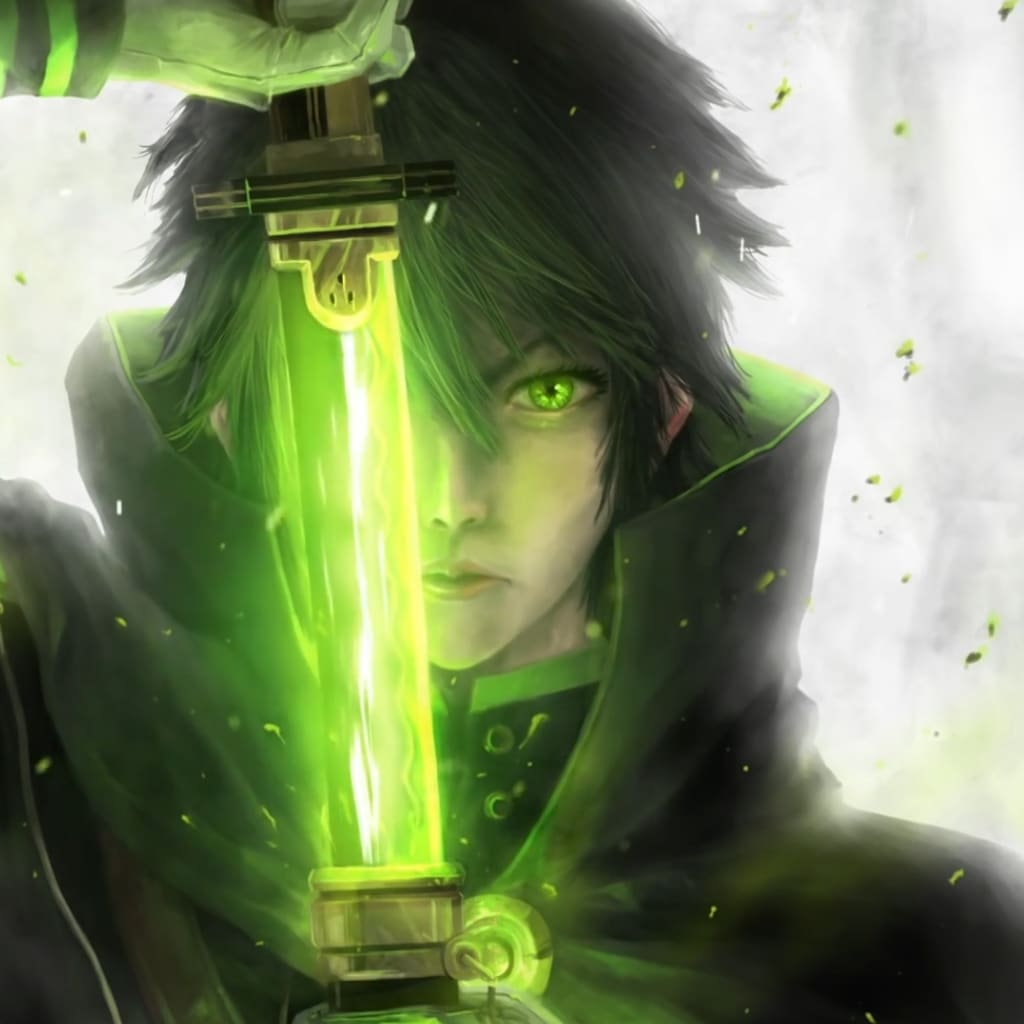# What Do Vampires And Numbers Have In Common? Count.

## The Real Origin Of Numbers

By ShanePublished 19 days ago 4 min read
LikeTo Understand Numbers Is To Understand Vampires

First I Will Show You How To Use Numbers To Spell Words

Then I Will Show You How It Works

The English Language Is Not A Human Language

Its Roman Roots Derive From Romania (Vampire History)

A = 1, B = 2, C = 3 D = 4 E = 5 F = 6 G = 7 H = 8 I = 9 J = 10 K = 11 L = 12

M = 13 N = 14 O = 15 P = 16 Q = 17 R = 18 S = 19 T = 20 U = 21 V = 22

W = 23 X = 24 Y = 25 Z = 26

Note: I Use "+" And "-" For Both Numbers And Words

"I Am A Vampire" = 9 + 1 13 + 1 + 22113169185

9113122113169185 = 3 (25 x 10 Primed) x 917515 x 2091471

"Demon King" = 45131514 + 119147

45131514119147 = (2402230003 + 444444444444) x 101

(All Numbers Used Are Connected To "Demon King", I Will Show You)

"The One Dark Lord" = 2085 + 15145 + 411811 + 1215184

2085151454118111215184 = 2035491 x (2 x 2 x 2 x 2 x 479 x 133663528841)

"The x One Dark Lord" = (33 x 34) + 34 + 49

11223449 = 23431 x 479

Vampire + Vampire Fangs Are Represented By "8220224"

84 = "Vampire" (22+1+13+16+9+18+5)

22 22 = "V V", The Vampire Fangs

Number 0 Represents The Vampire Mouth

8220224 = 8(2+3+1) x 4 x 23896

This Is Where I Tell You How The Numbers Work

By First Showing You I Am Using The Same Numbers

"The One Dark Lord" And "The x One Dark Lord" Are Both Using A 479

479 Is "7" Demon Horn + "49" Lord For "Demon Lord"

The 2 x 2 x 2 x 2 Following It Is V V (Vampire Fangs Of A Demon King)

The 133663528841 Reads Backwards "1", "84", "28", "12+54 35" "33 1"

"1" = A

"84" = Vampire

"28" = Man

1254 = "R"ed

35 = Eye

33 = The

1 = "One"

The Second "479" Is Followed By A "23431"

"479" And "23431" Reads "23" "1" "34" "49" + "7"

23 = I Am

1 = A

34 = Dark

49 = Lord

"7" = "Demon Horn"

"I Am A Vampire" Was 3 x (25 x 10 Primed) x 917515 x 2091471

3 25 10 Reads "23" "1" "3+2" Which Is "23" "1" "8x4" + 0 Mouth

23 = I Am 1 = A 84 = Vampire (I Used "I Am A Vampire" Twice)

(Note: 3 x (25 x 10 Primed) Is 4749)

The 917515 Reads As (3x3)1 + "7" + "51" + "41"

33 = The

1 = "One"

"7" = "Demon Horn"

51 = Demon

41 = King

The "2091" And "471" Read As "51 x 41" And "471" A Third Time

51 = Demon

41 = King

Therefore "2091" Is Demon x King

"471" Is "Demon Horn" + King

Finally, The "8220224" Vampire + Vampire Fangs + Mouth

Was 8(2+3+1) x 4 x 23896

23 1 84 = "I Am A Vampire" (9+1+13)+(1)+(22+1+13+16+9+18+5)

23896 Is "38" And A "296"

"296" Is Between 5141 And 51+41 (Demon King) (Demon+King)

5141 - 296 x (2+9+6) - (2+9+6) = 51+41

"38" Is "2 x 96" = 192 = 19 x 2 = "38"

Once Again, "The One Dark Lord" In Numbers:

"The One Dark Lord" And "The x One Dark Lord" Are Both Using A 479

479 Is "7" Demon Horn + "49" Lord For "Demon Lord"

The 2 x 2 x 2 x 2 Following It Is V V (Vampire Fangs Of A Demon King)

The 133663528841 Reads Backwards "1", "84", "28", "12+54 35" "33 1"

"1" = A

"84" = Vampire

"28" = Man

1254 = "R"ed

35 = Eye

33 = The

1 = "One"

The Second "479" Is Followed By A "23431"

"479" And "23431" Reads "23" "1" "34" "49" + "7"

23 = I Am

1 = A

34 = Dark

49 = Lord

"7" = "Demon Horn"

"I Am A Vampire" Was 3 x (25 x 10 Primed) x 917515 x 2091471

3 25 10 Reads "23" "1" "3+2" Which Is "23" "1" "8x4" + 0 Mouth

23 = I Am 1 = A 84 = Vampire (I Used "I Am A Vampire" Twice)

(Note: 3 x (25 x 10 Primed) Is 4749)

The 917515 Reads As (3x3)1 + "7" + "51" + "41"

33 = The

1 = "One"

"7" = "Demon Horn"

51 = Demon

41 = King

The "2091" And "471" Read As "51 x 41" And "471" A Third Time

51 = Demon

41 = King

Therefore "2091" Is Demon x King

"471" Is "Demon Horn" + King

how topop culturehumanityhistoryfeature
Like

How does it work?

There are no comments for this story

Be the first to respond and start the conversation.